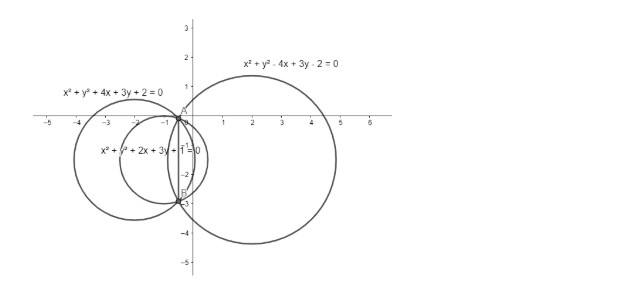Courses
Courses for Kids
Free study material
Free LIVE classes
MoreLIVE
Join Vedantu’s FREE Mastercalss

# Find the circle whose diameter is the common chord of the circles ${{x}^{2}}+{{y}^{2}}+2x+3y+1=0$ and ${{x}^{2}}+{{y}^{2}}+4x+3y+2=0$Verified
360.3k+ views
Hint: Write the equation of the common chord on the two circles and find the points of intersection of the two circles. Find the length of the chord, which is the diameter of the circle and the end point of chords can be used to find the centre of the circle. Write equation of circle using diameter of circle and its centre.

We have two circles whose equations are ${{S}_{1}}:{{x}^{2}}+{{y}^{2}}+2x+3y+1=0$ and ${{S}_{2}}:{{x}^{2}}+{{y}^{2}}+4x+3y+2=0$. We want to find the common chord of these two circles.We know that the equation of chords joining two circles of the form ${{x}^{2}}+{{y}^{2}}+2{{g}_{1}}x+2{{f}_{1}}y+{{c}_{1}}=0$ and ${{x}^{2}}+{{y}^{2}}+2{{g}_{2}}x+2{{f}_{2}}y+{{c}_{2}}=0$ is $2\left( {{g}_{1}}-{{g}_{2}} \right)x+2\left( {{f}_{1}}-{{f}_{2}} \right)y+{{c}_{1}}-{{c}_{2}}=0$.
Substituting ${{g}_{1}}=1,{{f}_{1}}=\dfrac{3}{2},{{g}_{2}}=2,{{f}_{2}}=\dfrac{3}{2},{{c}_{1}}=1,{{c}_{2}}=2$ in the above equation, we get $2\left( 1-2 \right)x+2\left( \dfrac{3}{2}-\dfrac{3}{2} \right)y+1-2=0$ as the equation of common chords of the given circle.
Thus, we have $2x+1=0$ as the equation of common chords.
We know that the equation of circle whose diameter is the common chord of the two given circles is ${{S}_{1}}+\lambda {{S}_{2}}=0$.
Thus, we have ${{x}^{2}}+{{y}^{2}}+2x+3y+1+\lambda \left( {{x}^{2}}+{{y}^{2}}+4x+3y+2 \right)=0$.
Now simplifying the above equation, we get ${{x}^{2}}\left( 1+\lambda \right)+{{y}^{2}}\left( 1+\lambda \right)+x\left( 2+4\lambda \right)+y\left( 3+3\lambda \right)+1+2\lambda =0$.
We know that the centre of this circle passes through the chord which is the diameter of this circle.
We know that the centre of circle with equation of the form ${{x}^{2}}+{{y}^{2}}+2gx+2fy+c=0$ is $\left( -g,-f \right)$
Thus, the centre of our circle ${{x}^{2}}\left( 1+\lambda \right)+{{y}^{2}}\left( 1+\lambda \right)+x\left( 2+4\lambda \right)+y\left( 3+3\lambda \right)+1+2\lambda =0$ is $\left( -\dfrac{2+4\lambda }{2},-\dfrac{3+3\lambda }{2} \right)$.
We know that this centre passes through the chord $2x+1=0$.
Substituting the centre of circle in equation of chord, we get $2\left( -\dfrac{2+4\lambda }{2} \right)+1=0$
On further solving, we get
\begin{align} & \Rightarrow -2-4\lambda +1=0 \\ & \Rightarrow 4\lambda =-1 \\ & \Rightarrow \lambda =\dfrac{-1}{4} \\ \end{align}
Substituting the value $\lambda =\dfrac{-1}{4}$ in the equation of circle ${{x}^{2}}\left( 1+\lambda \right)+{{y}^{2}}\left( 1+\lambda \right)+x\left( 2+4\lambda \right)+y\left( 3+3\lambda \right)+1+2\lambda =0$, we get ${{x}^{2}}\left( 1+\dfrac{-1}{4} \right)+{{y}^{2}}\left( 1+\dfrac{-1}{4} \right)+x\left( 2+4\left( \dfrac{-1}{4} \right) \right)+y\left( 3+3\left( \dfrac{-1}{4} \right) \right)+1+2\left( \dfrac{-1}{4} \right)=0$.
On further solving, we have $\dfrac{3{{x}^{2}}}{4}+\dfrac{3{{y}^{2}}}{4}+x+\dfrac{9y}{4}+\dfrac{1}{2}=0$.
Hence, we get $3{{x}^{2}}+3{{y}^{2}}+4x+9y+2=0$ as the equation of circle whose diameter is the common chord of the circles ${{S}_{1}}:{{x}^{2}}+{{y}^{2}}+2x+3y+1=0$ and ${{S}_{2}}:{{x}^{2}}+{{y}^{2}}+4x+3y+2=0$.

Note: One needs to know the formula for writing the equation of the common chord of two circles. Otherwise, we can also solve this question by solving the equation of two circles to find the common chord. One must carefully perform the calculations; otherwise, you will get an incorrect answer.

Last updated date: 15th Sep 2023
Total views: 360.3k
Views today: 10.60k